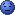CodeGuru Home VC++ / MFC / C++ .NET / C# Visual Basic VB Forums Developer.com

# Thread: How to open different variable file in excel application?

1.Junior MemberJoin Date
Jul 2012
Posts
7

## How to open different variable file in excel application?

Sorry, I'm beginner in VB6.
Now I want to open 2 kind of excel file.
First I define them A-File & B-File.
The A-file whose path is
Code:
`Open(App.Path & "\" & "Convert Excel.xls")`
But B-file which is variable file.
(I cant assure how many B-file)
I decide to use function in Module1 to control it.
Define global variable in module
Code:
```Public op As Boolean
Public optime As Integer
Public singlefile(500) As String```
This is openfile as B-File
Code:
```Private Sub Open_Single_File_Click()
CommonDialog1.DialogTitle = "Open Single File"
CommonDialog1.Filter = "Machine File(*.txt)|*.txt|All File(*.*)|*.*"
CommonDialog1.Action = 1
Text2.Text = CommonDialog1.FileName
op = True
optime = optime + 1
singlefile(optime) = Text2.Text
Text1.Text = "Open Type -> Single = " & optime
End Sub```
Creating open multiple excel file function
Code:
```Public Function openexcel(ByVal optime_ As Integer, ByVal singlefile_ As String)
Dim xlapp As Excel.Application
Dim xlBook As Excel.Workbook
Dim xlSheet As Excel.Worksheet
Set xlapp = CreateObject("Excel.Application")
Set xlBook = xlapp.Workbooks.Open(singlefile_)
xlapp.Visible = False

End Function```
Another Excel button
Code:
```Private Sub Command2_Click()
cho = MsgBox("It must to have Excel,Do your computer have Excel?", vbOKCancel, "Caution")
If cho = vbOK Then
If op = True Then

Dim xlapp As Excel.Application
Dim xlBook As Excel.Workbook
Dim xlSheet As Excel.Worksheet
Set xlapp = CreateObject("Excel.Application")
Set xlBook = xlapp.Workbooks.Open(App.Path & "\" & "Convert Excel.xls")
xlapp.Visible = False
For l = 1 To optime    '<--this have bug
Dim xlapp(l) As Excel.Application '<---This have but
xlapp(l) = openexcel(l, singlefile(l)) '<---this have but
Next l
Else
b = MsgBox("The file have not open yet!", vbOKOnly, "Caution")
End If
Else
End If
End Sub```Reply With Quote

2. ## Re: How to open different variable file in excel application?

There are a couple of mistakes you made:
a) array indexes start at 0 (except you have stated otherwise)
Code:
```op = True
singlefile(optime) = Text2.Text 'first set element 0
optime = optime + 1 'then increment counter```
Also the for loop within Command2_Click() must start at 0
Code:
```  Fo l=0 to optime-1
...```
b) as you did not dim xlapp as an array, so xlapp(l) will invariably be an error. You could
Code:
```
Dim xlapp() as Excel.Application
...
For l = 0 to optime - 1
ReDim xlapp(l)
set xlapp(l) = openexcel(l, singlefile(l))
Next```
But this is only hinting the obvious technical errors. We do not know what you want to achieve exactly. Therefore there may be more flaws within your concept which are not as easy to spot.

optime = optime + 1Reply With Quote

3.Super Moderator Power PosterJoin Date
Jul 2008
Location
WV
Posts
5,348

## Re: How to open different variable file in excel application?

Actually that redim should be outside the loop, once you know the value of optime then you know how big the array needs to be so woudl be better to do it once rather than with every loop also the value is going to be lost with each redim so it would need to be redim preserve if inside the loop and that would be even slower.
Code:
```Dim xlapp() as Excel.Application
...
ReDim xlapp(optime)

For l = 0 to optime - 1
set xlapp(l) = openexcel(l, singlefile(l))
Next```Reply With Quote

####Posting Permissions

• You may not post new threads
• You may not post replies
• You may not post attachments
• You may not edit your posts
•